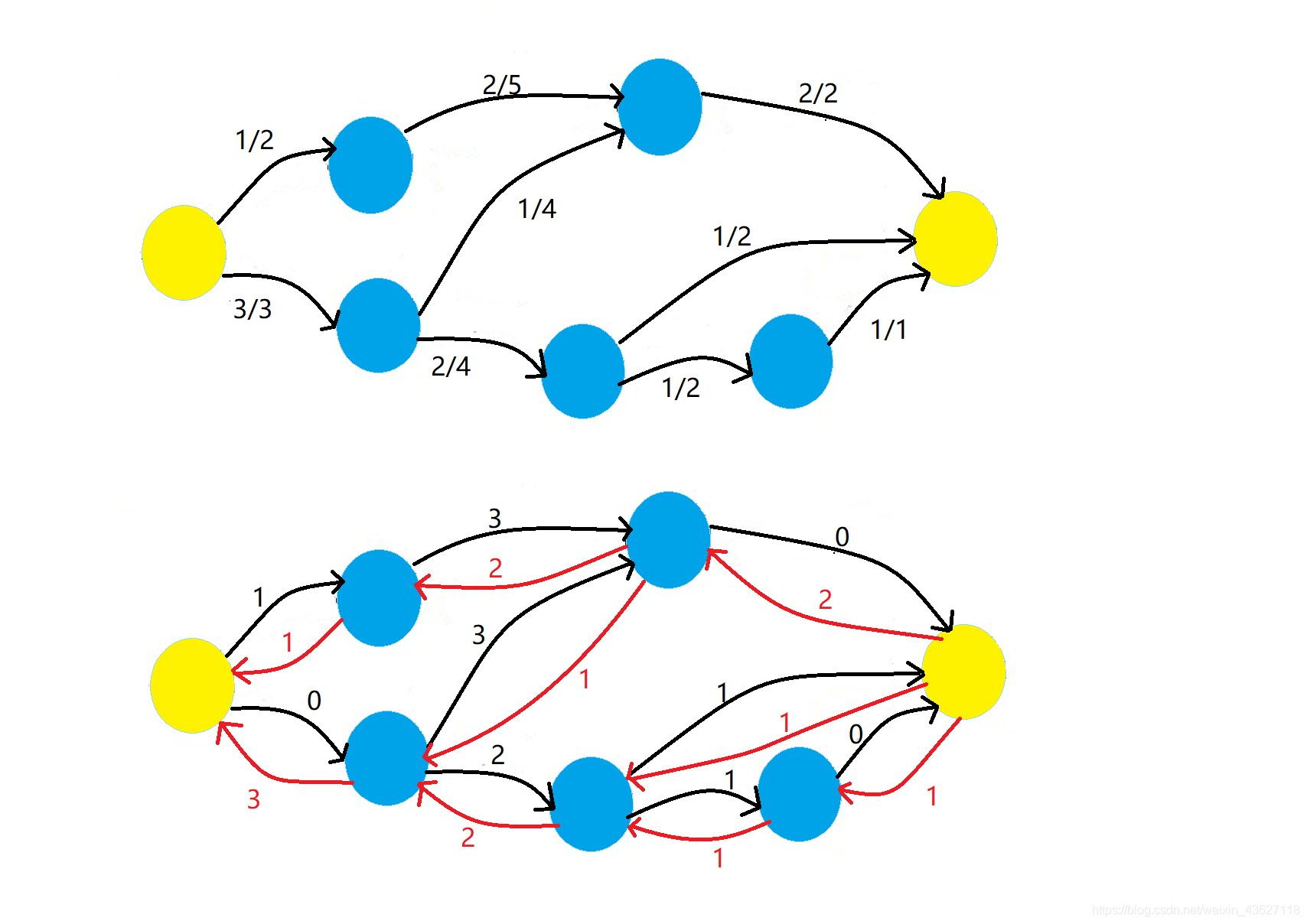# 图论 -- 网络流最大流问题Edmonds-Karp 算法：以广度优先的增广路算法，又称为最短增广路算法（Shortest Augument Panth, SAP）。

1. 初始化可行流 flow 为零流，即实流网络中全是零流边，残余网络中全是最大容量边（可增量）。初始化 vis[]数组为 false，pre[]数组为−1。

2. 令 vis[s]=true，s 加入队列 q。

3. 如果队列不空，继续下一步，否则算法结束，找不到可增广路。当前的实流网络就是最大流网络，返回最大流值 maxflow。

4. 队头元素 new 出队，在残余网络中检查 new 的所有邻接结点 i。如果未被访问，则访问之，令 vis[i]=true，pre[i]=new；如果 i=t，说明已到达汇点，找到一条可增广路，转向第（5）步；否则结点 i 加入队列 q，转向第（3）步。

5. 从汇点开始，通过前驱数组 pre[]，逆向找可增广路上每条边值的最小值，即可增量 d。

6. 在实流网络中增流，在残余网络中减流，Maxflow+=d，转向第（2）步。

这里给出 EK 算法模板：

#include <iostream>#include <cstdio>#include <cstring>#include <cmath>#include <algorithm>#include <vector>#include <set>#include <map>#include <queue>#define INF 0x3f3f3f3fusing namespace std;int n,m;const int maxn=1005;int g[maxn][maxn];//容量int f[maxn][maxn];//流量bool vis[maxn];int pre[maxn];bool bfs(int s,int t){    memset(pre,-1,sizeof pre);    memset(vis,false,sizeof vis);    queue<int> q;    vis[s]=true;    q.push(s);    while(!q.empty()){        int now=q.front();        q.pop();        for(int i=1;i<=n;i++){            if(!vis[i]&&g[now][i]){                vis[i]=true;                pre[i]=now;                if(i==t) return true;                q.push(i);            }        }    }    return false;}int EK(int s,int t){    int maxflow=0;    int u,v;    while(bfs(s,t)){        int d=INF;        v=t;        while(v!=s){            u=pre[v];            if(d>g[u][v])                d=g[u][v];            v=u;        }        maxflow+=d;        v=t;        while(v!=s){            u=pre[v];            g[u][v]-=d;            g[v][u]+=d;            if(f[v][u]>0)                f[v][u]-=d;            else f[u][v]+=d;            v=u;        }    }    return maxflow;}int main(){    //int s,t;    int u,v,c;    while(~scanf("%d%d",&m,&n)){        memset(f,0,sizeof f);        memset(g,0,sizeof g);        for(int i=1;i<=m;i++){            scanf("%d%d%d",&u,&v,&c);            g[u][v]+=c;        }        printf("%d\n",EK(1,n));    }    return 0;}## 评论